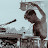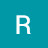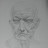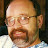Square and Cube Roots 4 Rationalize Denominator – Tutor AlgebraSquare and Cube Roots 4 Rationalize Denominator – Tutor Algebra

This math video tutors and gives instruction about square and/or cube roots. The instruction is a brief and to the point teaching lesson on how to learn algebra and manipulate variables.
Square roots and cube roots are tricky to simplify and understand. This video is part of a series of videos that starts with simple problems and progresses to explain even the most difficult of problems that involve square roots and cube roots.

algebra tutors,algebra,college,cube,educational,equation,exponent,expression,for,ged,help,high,homework,how to,inequality,instruction,learn,lesson,linear,math,mathematics,online,practice,pre,property,pythagorean,root,school,simplify,solve,solving,square,system,teach,theorem,tutor,tutorial,tutoring,variable,video,with

algebratutors.org

4 Responses to “Square and Cube Roots 4 Rationalize Denominator – Tutor Algebra”

1.Quantum Reality says:

Your doing too simple problems , but the method is quite good and I think you can go for some advanced problems

2.Rebecca Riehl says:

The video says cubed roots as well….but no cube roots are completed.

3.Panamaniacs 2011 says:

great video!! tanx!! could u make a tutorial with cubes and beyond. because i have problems to identify what number i have to multiply when is a cubic root specially with variables. tanx

4.Paul Bogdan says:

You're welcome. If you need any help, drop me a line. I liked the Ralphie May video, thank you.